Get inspired by the success stories of our students in IIT JAM MS, ISI  MStat, CMI MSc DS.  Learn More

# ISI MStat PSB 2004 Problem 7 | Finding the Distribution of a Random VariableThis is a very beautiful sample problem from ISI MStat PSB 2004 Problem 7 based on finding the distribution of a random variable . Let's give it a try !!

## Problem- ISI MStat PSB 2004 Problem 7

Suppose X has a normal distribution with mean 0 and variance 25 . Let Y be an independent random variable taking values -1 and 1 with equal probability. Define S=XY+XY$S=X Y+\frac{X}{Y}$ and T=XYXY$T=X Y-\frac{X}{Y}$
(a) Find the probability distribution of s.
(b) Find the probability distribution of (S+T10)2$(\frac{S+T}{10})^{2}$

### Prerequisites

Cumulative Distribution Function

Normal distribution

## Solution :

(a) We can write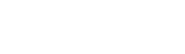Let Cumulative distribution function of S be denoted by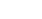. Then ,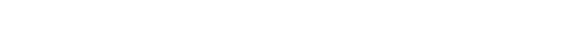----(1)

Here given that Y takes values 1 and -1 with equal probabilities .so ,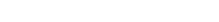.

Now as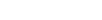hence X is symmetric distribution about 0 . Thus X and -X are identically distributed .

Thus from (1) we get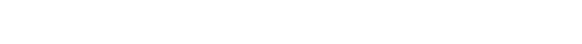=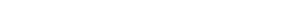Hence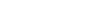.

(b) Let K=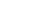=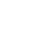Let C.D.F of K be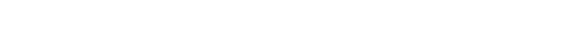=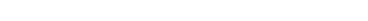as.

## Food For Thought

Find the distribution of T .

## Subscribe to Cheenta at Youtube

This is a very beautiful sample problem from ISI MStat PSB 2004 Problem 7 based on finding the distribution of a random variable . Let's give it a try !!

## Problem- ISI MStat PSB 2004 Problem 7

Suppose X has a normal distribution with mean 0 and variance 25 . Let Y be an independent random variable taking values -1 and 1 with equal probability. Define S=XY+XY$S=X Y+\frac{X}{Y}$ and T=XYXY$T=X Y-\frac{X}{Y}$
(a) Find the probability distribution of s.
(b) Find the probability distribution of (S+T10)2$(\frac{S+T}{10})^{2}$

### Prerequisites

Cumulative Distribution Function

Normal distribution

## Solution :

(a) We can writeLet Cumulative distribution function of S be denoted by. Then ,----(1)

Here given that Y takes values 1 and -1 with equal probabilities .so ,.

Now ashence X is symmetric distribution about 0 . Thus X and -X are identically distributed .

Thus from (1) we get=Hence.

(b) Let K==Let C.D.F of K be=as.

## Food For Thought

Find the distribution of T .

## Subscribe to Cheenta at Youtube

This site uses Akismet to reduce spam. Learn how your comment data is processed.

### Knowledge Partner